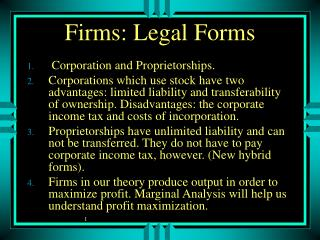DownloadDownload PresentationFirms: Legal Forms

Firms: Legal Forms

Download PresentationFirms: Legal Forms

- - - - - - - - - - - - - - - - - - - - - - - - - - - E N D - - - - - - - - - - - - - - - - - - - - - - - - - - -
Presentation Transcript

1. Firms: Legal Forms • Corporation and Proprietorships. • Corporations which use stock have two advantages: limited liability and transferability of ownership. Disadvantages: the corporate income tax and costs of incorporation. • Proprietorships have unlimited liability and can not be transferred. They do not have to pay corporate income tax, however. (New hybrid forms). • Firms in our theory produce output in order to maximize profit. Marginal Analysis will help us understand profit maximization.

2. Marginal Analysis • Relationship between Total, Average, and Marginal Magnitudes? • You already have experience – you have been calculating your ‘average’ since elementary school. Each test is a ‘marginal’ score. • Useful in Understanding Profit Maximization. • Total Revenue is defined as Price multiplied by Quantity. • MR is the change in TR when another unit is sold.

3. Marginal Analysis - Demand • Note Relationship between elasticity and Marginal Revenue.

4. More Marginal Analysis

5. Marginal Costs and Profit

6. Profit Maximization • Marginal Analysis • TR= PxQ • Calculus leads to MR=MC conclusion; • Alternatives to Calculus • AR = demand curve; marginal revenue curve must lie below demand curve • Profit maximized when TR-TC is greatest (vertical difference) • this implies slope of TR = slope of TC which means that MR=MC

7. Some simple Calculus p = - TR TC ¶p ¶ ¶ TR TC = - = - MR MC ¶ ¶ ¶ Q Q Q ¶p p Þ = Max 0 ¶ Q = so MR MC Also = · TR P Q ¶ ¶ ¶ TR Q P = · + · P Q ¶ ¶ ¶ Q Q Q ¶ Q P 1 = + · = - P ( 1 ) P ( 1 ) ¶ e P Q e = where elasticity of demand

8. The Intuition = D Marginal Revenue in Revenue when Q + 1 units are sold instead of Q units. Last Unit brings revenues = price of last unit + D · P Q for Q units which go down in price. D MR is less than P because of this P effect. D · D So MR = P - ( P Q) where Q = 1 D · P Q 1 = - MR = P(1 - ) or MR P ( 1 ) where D · e Q P e = elasticity of demand

9. Profit Maximization TC \$ TR D max Rev q* Max Profit Output MR

10. Another Angle AC MC P* Profits D q* MR

11. The Firm's Inputs And Costs • Fixed And Variable Costs. • Fixed Costs: Costs that do not change when output changes. • Variable costs: Costs that do change when output changes. • Long Run and Short run. • Long Run: A long enough period of Time such that all costs are variable • Short Run: A period of time such that at least one input (cost) is fixed.

12. Total Fixed Total Fixed TC=FC+VC; TC/Q = FC/Q +VC/Q which is ATC= AFC +AVC Fixed and Variable Costs AC P AVC AFC Q q1

13. Irrelevance of Fixed Costs if you stay in Business • Changes in Fixed costs don't alter profit maximizing P and Q because fixed Costs don’t impact Marginal Costs. • Fixed Costs do impact profits, and may cause firm to decide the leave industry. • Same with lump sum taxes.

14. Impact of Fixed Costs on Profit AC2 AC1 MC P* Profits2 AC* Profits1 AC** D MR q*

15. Economies and Diseconomies of Scale • What does this imply about the AC curve? • defined simply as whether or not AC rises or falls • long run AC Vs. short run AC • Distinguishing between economies of scale and improvements in technology very important. • Can firms have diseconomies of scale but industries have economies of scale?

16. AC Economies of Scale Diseconomies of Scale

17. Long Run AC LRAC SRAC1 MC1 SRAC3 MC3 MC2 SRAC2 Q1

18. Do average costs fall over time, or is average cost downward sloping? AC1990 AC1991 P* AC1992 AC1993 AC1994 q*

19. Can firms have diseconomies of scale but industries have economies of scale? • External Effects – Industry output effects the costs of individual firms. • Positive External Effects can cause AC for industry to fall even though each firm has upward sloping AC curve. • Used to explain apparent decreasing costs but multiple firms in industry.